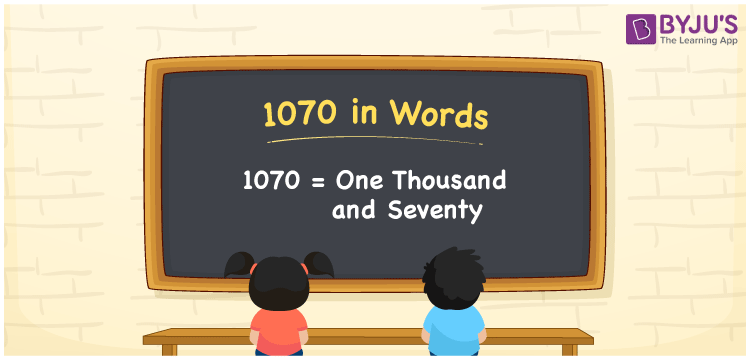# 1070 in Words

1070 in words is represented by One Thousand and Seventy. For instance, if you buy a bicycle worth Rs. 1070, then you can say, “I bought a bicycle worth Rupees One Thousand and Seventy”. 1070 is a cardinal number since it depicts a specific value. Learn the tricks of converting the number 1070 in word form with the help of a place value table here.

 1070 in Words One Thousand and Seventy One Thousand and Seventy in numerical form 1070

## 1070 in English Words

We usually write the numbers in words using the letters of the English alphabet. Hence, we can read the number 1070 in words as One Thousand and Seventy.## How to Write 1070 in Words?

The place value chart for the number 1070 is as follows.

 Thousands Hundreds Tens Ones 1 0 7 0

Therefore, we can write the expanded form as:

1 x Thousand + 0 x Hundred + 7 x Ten + 0 x One

= 1 x 1000 + 0 x 100 + 7 x 10 + 0 x 1

= 1000 + 0 + 70 + 0

= 1000 + 70

= 1070

= One Thousand and Seventy

Hence, 1070 in words is written as One Thousand and Seventy

Interesting way of writing 1070 in words

1 = One

10 = Ten

107 = One Hundred and Seven

1070 = One Thousand and Seventy

Thus, the word form of the number 1070 is One Thousand and Seventy

1070 is a natural number that is one less than 1071 and one greater than 1069

• 1070 in words – One Thousand and Seventy
• Is 1070 an odd number? – No
• Is 1070 an even number? – Yes
• Is 1070 a perfect square number? – No
• Is 1070 a perfect cube number? – No
• Is 1070 a prime number? – No
• Is 1070 a composite number? – Yes

## Frequently Asked Questions on 1070 in Words

Q1

### How to write 1070 in words?

We can write 1070 in words as One Thousand and Seventy.
Q2

### What is the place value of 7 in 1070?

The place value of 7 in 1070 is Tens.
Q3

### Is 1070 a composite number?

Yes, the number 1070 is a composite number.